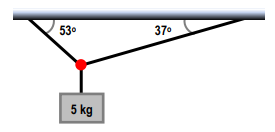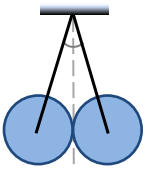Ch 05: Intro to Forces (Dynamics)WorksheetSee all chapters
 Ch 01: Intro to Physics; Units 1hr & 22mins 0% complete Worksheet Ch 02: 1D Motion / Kinematics 4hrs & 13mins 0% complete Worksheet Ch 03: Vectors 2hrs & 2mins 0% complete Worksheet Ch 04: 2D Motion (Projectile Motion) 3hrs & 8mins 0% complete Worksheet Ch 05: Intro to Forces (Dynamics) 3hrs & 42mins 0% complete Worksheet Ch 06: Friction, Inclines, Systems 4hrs & 32mins 0% complete Worksheet Ch 07: Centripetal Forces & Gravitation 3hrs & 51mins 0% complete Worksheet Ch 08: Work & Energy 3hrs & 56mins 0% complete Worksheet Ch 09: Conservation of Energy 7hrs & 4mins 0% complete Worksheet Ch 10: Momentum & Impulse 5hrs & 35mins 0% complete Worksheet Ch 11: Rotational Kinematics 3hrs & 4mins 0% complete Worksheet Ch 12: Rotational Inertia & Energy 7hrs & 7mins 0% complete Worksheet Ch 13: Torque & Rotational Dynamics 2hrs & 9mins 0% complete Worksheet Ch 14: Rotational Equilibrium 4hrs & 10mins 0% complete Worksheet Ch 15: Angular Momentum 3hrs & 6mins 0% complete Worksheet Ch 16: Periodic Motion 2hrs & 17mins 0% complete Worksheet Ch 17: Waves & Sound 3hrs & 25mins 0% complete Worksheet Ch 18: Fluid Mechanics 4hrs & 39mins 0% complete Worksheet Ch 19: Heat and Temperature 4hrs & 9mins 0% complete Worksheet Ch 20: Kinetic Theory of Ideal Gasses 1hr & 40mins 0% complete Worksheet Ch 21: The First Law of Thermodynamics 1hr & 49mins 0% complete Worksheet Ch 22: The Second Law of Thermodynamics 4hrs & 56mins 0% complete Worksheet Ch 23: Electric Force & Field; Gauss' Law 3hrs & 32mins 0% complete Worksheet Ch 24: Electric Potential 1hr & 55mins 0% complete Worksheet Ch 25: Capacitors & Dielectrics 2hrs & 2mins 0% complete Worksheet Ch 26: Resistors & DC Circuits 3hrs & 20mins 0% complete Worksheet Ch 27: Magnetic Fields and Forces 2hrs & 35mins 0% complete Worksheet Ch 28: Sources of Magnetic Field 2hrs & 30mins 0% complete Worksheet Ch 29: Induction and Inductance 3hrs & 38mins 0% complete Worksheet Ch 30: Alternating Current 2hrs & 37mins 0% complete Worksheet Ch 31: Electromagnetic Waves 1hr & 12mins 0% complete Worksheet Ch 32: Geometric Optics 3hrs 0% complete Worksheet Ch 33: Wave Optics 1hr & 15mins 0% complete Worksheet Ch 35: Special Relativity 2hrs & 10mins 0% complete Worksheet Ch 36: Particle-Wave Duality Not available yet Ch 37: Atomic Structure Not available yet Ch 38: Nuclear Physics Not available yet Ch 39: Quantum Mechanics Not available yet

# 2D Equilibrium

See all sections

Concept #1: 2D Equilibrium Problems

Transcript

Hey guys so in this video I want to talk about two dimensional work equilibrium problems which are problems where forces are gonna cancel but at least one force is going to be an angle not saying flatten on the x and the y but at an angle so when we cancel all the forces we have to do this both in the x and the y axis. Let me show you. So the first thing we're going to have to do is decompose all the forces because like I said at least one of the forces will be at an angle and then because we're in equilibrium in both dimensions I can say that the sum of all forces in the x equals zero and the sum of all forces in the y equals zero. Ok So, here I have let me call this T1 and I can call this T2. The other forces acting here are there's actually one more actually mg and just real quick I'm gonna use gravity as as 10 instead of 9.8 just around to 50. And this T2 here has to be decomposed into T2(x) and T2(y). When I do this I want the angle not to be up here but I want the angle to be down here. However it turns out that this is exactly the same angle because this reflects over here so 37 is the angle that I would use. So I set up the sum of all forces equals zero in the x axis and the y axis. But the way that I like to think of these problems that I think makes more sense it's more visual it's to think that's in these statements are correct that all the forces to the left equal all the forces to the right because all the forces pulling this way equal all the forces pulling this way. And we've done this before. Same thing here all the forces up equals all the forces now. And also I have absolute value signs here. And the reason for that is that when I write this way I don't have to worry about signs. And I'll show why really quick. So I'm going to start with sum of our forces in the x axis equal zero. And there are only two forces in the x axis T1 and T2(x) so you could do it this way you could say T1 is to the left so I'm gonna make it negative T1 plus T2(x) to the right so I'm gonna make it positive T2(x) equals zero. And then I'm going to move T1 to the other side of the equation so that it becomes positive and then I get T2(x) equals T1, T2(x) is a force on the right and T1 is the force on the left. So left equals right and they're both positive. But instead of going through all of this I could have just looked at the drawing here and said Hey these two have to be the same. Because this is at equilibrium and I would have arrived at this much faster. But notice that they're both positive because magnitudes are the same. So I can do this in the y axis as well. And then this would mean that this equals this. Sum of all forces in y equals zero. But I'm going to skip some steps here and just say top equals bottom. So you want to write this you want to say left cancels with right, up cancels with down and then we start trying to solve this. So if you go here I don't have T1 and I don't know T2(x) either but I can expand T2(x) into T2 cosine of theta now you always want to do this you only want to expand. On a component of a vector if you know at least one of these two variables. Right now I know zero out of two variables. And if I expand I know one out of three variables and that's better. Even though it miight not seem better because I'm still missing two things. I'm in fact a little closer so. What you don't want to do is expand this. If you don't know any of these three then you will know zero out of three which is certainly worse than zero out of two things. OK. So if you know one of the two you want to expand and I know this. So I can write T1 equals T2 cosine of 37, cosine of 37 is point eight so T1 equals Point eight T2, I'm stuck here I can go anywhere else. So I'm gonna have to continue over here and hopefully the y axis workout. And I do know mg I know mg is 50. And again because I know. One of the two. Variables that T2(y) can be changed into T2 sine of theta I know theta then I'm going to replace I'm gonna expand T2(y) so T2 Sine of 37 equals 50 if I move things around T2 I find to be eighty three point three. That's the first answer. And obviously to get the second answer you just have to plug this back here. So T1 is point eight times eighty three point three which is sixty six point seven Newtons and that is my second answer. Alright so you gonna say left equals right top equals down trying to solve for what you're looking. Let's check out the next one. So here I have a 1 metre long string connected to the center of a two kilogram ball. So the length of the string is this. The mass of the ball is two kilograms put over here. And it is 30 centimeters in diameter so diameter equals point three meters, remember in physics we're only going to use radius so let me convert that real quick. Which rests against a smooth. This means no friction vertical wall and shown. So I want to know tension in the string the force of the wall on the ball. So let me draw a free body diagram here real quick, let me draw these forces here real quick first. So I got a T here. Tx here. And we got a Ty here. There's also an mg here. OK.

So I drew a T it's an angle. Ideally I want this angle to be down here and I can split it up into Tx and Ty those are sort of the obvious forces and at this point you might wonder is there anything else. Well this ball is being pulled to the right by the tension because of the way that's it is hanging T gets decomposed into Tx and Ty so we got a Tx in the right which means the ball is pushing against the wall therefore the wall has to push back against the wall. Right.